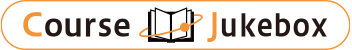### CourseDetailDegree
Bachelor
1
Course delivery methods
face-to-face
Subject
Physical sciences, Mathematical sciences, Engineering & technology
Program
School
School of Science and Engineering
Department
Bachelor's Program in Interdisciplinary Engineering
Campus
Tsukuba Campus
Classroom
3B303
Course Offering Year
2023-2024
Course Offering Month
April - May
April - August
Weekday and Period
Tue5,6 Thu4,5
Capacity
Credits
4.0
Language
English
Course Number
FJ20144

### Advanced Calculus University of Tsukuba

#### Course Overview

Following“Introduction to Single-Variable Calculus I & II," this course introduces the basic tools of calculus and develops their technical competence, namely, differential equations, infinite series, vector calculus, curvilinear coordinate systems, and partial derivatives, etc. This is achieved by visualization, numerical and graphical experimentations and, thus, students are required to be acquainted with Mathematica (or similar ones) during the course as working exercises and homework problems. This course as well as “Introduction to Single-Variable Calculus I & II” provides a core and practical knowledge required for many courses in both natural and social sciences.

#### Learning Achievement

1. The students are able to express lines and planes in high dimensions in terms pf parameters.
2. The students are able to describe the conic sections by cartesian and polar coordinates.
3. The students are able to describe the geometry with vectors and vector functions.
4. The students are able to carry out partial derivatives of multi-variable functions.
5. The students are able to evaluate areas and volumes by multiple integrals.
6. The students are able to carry out derivatives and integrals of vector functions.

#### Competence

Related to 1. Mathematical logic and calculation skills.

#### Course prerequisites

* Students are required to have taken "Introduction to Single-Variable Calculus I and II" or equivalent courses.
If not, they are asked to discuss with the instructor before registering this course.
* Those who already earned the credit of Calculus II (FJ20114) are not allowed to earn the credit of this course.

Class performance 20%
Midterm 40%
Final 40%

#### Course schedule

Parametric Equations and Polar Coordinates
Conic Sections
Conic Sections in Polar Coordinates
Vectors and Geometry of Space
Vector Functions: dot product and cross product
Vector Functions: tangent vector, curves, derivatives, integrals
Summary and Recitation
Partial Derivatives: geometric interpretation
Partial Derivatives: implicit differentiation
Partial Derivatives: steepest decent, Lagrange multiplier
Mid-term Exam
Multiple Integrals: double integrals
Multiple Integrals: double integrals with general R
Multiple Integrals: triple integrals
Multiple Integrals: change of variables
Vector Calculus: line integrals
Vector Calculus: Green's theorem, vector operations
Vector Calculus: parametric surfaces
Vector Calculus: Gauss theorem and Stokes theorem
Summary and Recitation

#### Course type

Lectures and Class Exercises